# Dataset¶

Ascend GPU CPU 入门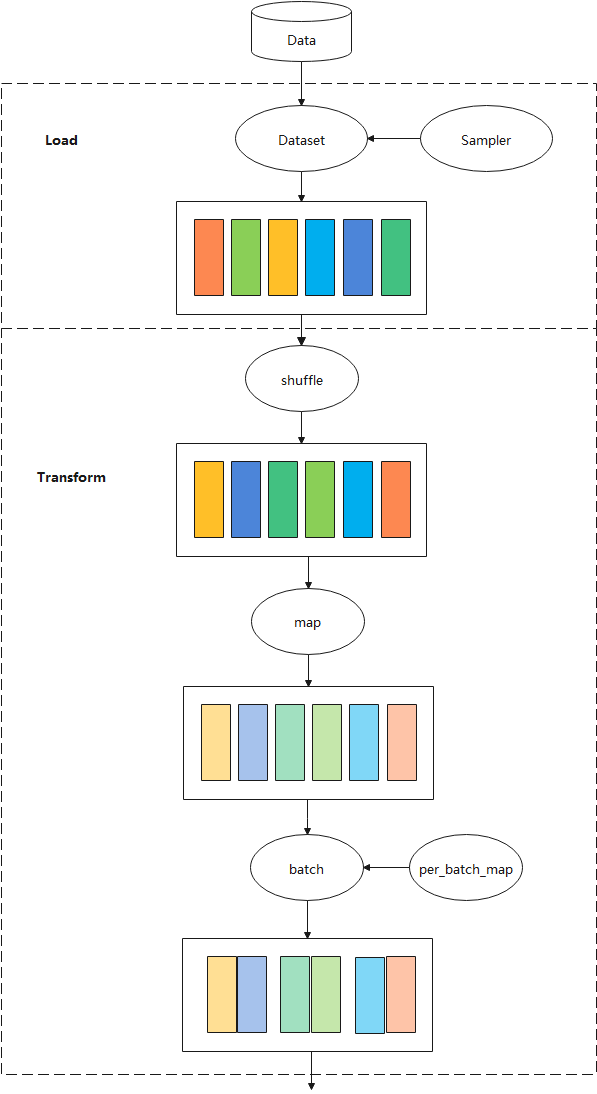## 数据集¶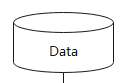Dataset支持多种格式数据集，包括MindSpore自研数据格式MindRecord，常用的公开图像数据集和文本数据集，用户自定义的数据集等。 详细的Mindspore支持的数据集请参考: MindSpore支持数据集

Dataset也支持将常用的数据集和用户自定义的数据集转为MindSpore数据格式（MindRecord），详情可参考： 转换数据集为MindRecord

## 数据集加载¶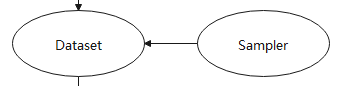Dataset对多种常用的数据集提供对应的类来实现数据集的加载，同时对于不同存储格式的数据文件，Dataset也有对应的类来进行数据加载。MindSpore数据集加载请参考：MindSpore数据集加载

Dataset提供了多种用途的采样器（Sampler），采样器负责生成读取的index序列，Dataset负责根据index读取相应数据，帮助用户对数据集进行不同形式的采样，以满足训练需求，解决诸如数据集过大或样本类别分布不均等问题，注意，采样器负责对样本做filter和reorder操作，不会执行Batch操作。

Mindspore的数据采样介绍请参考：MindSpore数据采样

## 数据处理¶

Dataset将数据加载到内存后，数据按Tensor形式进行组织。同时Tensor也是数据增强操作中的基本数据结构。

### Shuffle操作¶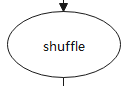Dataset提供多种方式来实现全局shuffle操作。

1. 数据集加载类的shuffle参数

import numpy as np
import mindspore.dataset as ds
data = [1, 2, 3, 4]
dataset = ds.NumpySlicesDataset(data=data, column_names=["column_1"], shuffle=True)


详情请参考：NumpySlicesDataset

2. shuffle算子

import numpy as np
import mindspore.dataset as ds
data = [1, 2, 3, 4]
dataset = ds.NumpySlicesDataset(data=data, column_names=["column_1"])
# buffer_size equal to the number of rows in the entire dataset will result in a global     shuffle
dataset = dataset.shuffle(4)


详情请参考：shuffle API

3. 随机采样

import numpy as np
import mindspore.dataset as ds
data = [1, 2, 3, 4]
sampler = ds.RandomSampler()
dataset = ds.NumpySlicesDataset(data=data, column_names=["column_1"],sampler=sampler)


详情请参考：RandomSampler

### Map操作¶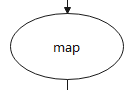Map操作对各类数据做数据增强，负责启动和执行Dataset提供或用户自定义的数据增强算子，对数据进行映射变换，其中数据增强是一种创造有着不同方向的“新”数据的方法，一是从有限数据中生成“更多数据”，二是防止过拟合。

Dataset的c_transformspy_transforms模块分别提供了基于C++Python的数据增强算子实现，同时用户可以自定义函数来进行数据增强。

Map操作请参考：Map操作

### Batch操作¶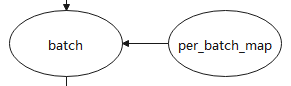Batch 操作负责将多个shape相同的Tensor“打包”到一起，以实现以mini-batch的方式来进行训练，Batch操作还提供drop_remainder参数，表示把最后一个不足batch_size的batch删除，默认会保留。假如数据集大小为17373，使用8张卡进行训练并且Batch size为16，每张卡分配2172条样本，当drop_remainder为True时，每张卡上可打包135个mini-batch。

import numpy as np
import mindspore.dataset as ds
# col1d: ,
# col2d: [,], [,]
def gen_2cols(num):
for i in range(num):
yield (np.array([i]), np.array([[i + 100], [i + 200]]))

dataset = ds.GeneratorDataset((lambda: gen_2cols(2)), ["col1d", "col2d"])
dataset = dataset.batch(batch_size=2, drop_remainder=False, pad_info={"col2d": ([2, 2], -2) , "col1d": (, -1)})
# col1d: [0, -1], [1, -1]
# col2d: [[100, -2], [200, -2]], [[101, -2], [201, -2]]

2. per_batch_map操作

import numpy as np
import mindspore.dataset as ds
# first column: 0, 3, 6, 9 ...
# second column:1, 4, 7, 10 ...
# third column: 2, 5, 8, 11 ...
def gen_3_cols(num):
for i in range(num):
yield (np.array([i * 3]), np.array([i * 3 + 1]), np.array([i * 3 + 2]))
# first epoch batch_size per batch: 1, 2 ,3 ...
# second epoch batch_size per batch: 2, 4, 6 ...
# third epoch batch_size per batch: 3, 6 ,9 ...
def batch_func(batchInfo):
return (batchInfo.get_batch_num() + 1) * (batchInfo.get_epoch_num() + 1)

# multiply first col by batch_num, multiply second col by -batch_num
def map_func(col1, col2, batchInfo):
return ([np.copy((1 + batchInfo.get_batch_num()) * arr) for arr in col1],
[np.copy(-(1 + batchInfo.get_batch_num()) * arr) for arr in col2])
# col1: [], [[ 6], ], []
# col2: [[-1]],[[ -8], [-14]],  [[-30]]
# col3: [], [, ], []
dataset = ds.GeneratorDataset((lambda: gen_3_cols(4)), ["col1", "col2", "col3"]).batch (batch_size=batch_func, input_columns=["col1", "col2"], per_batch_map=map_func)


Batch操作请参考：Batch操作